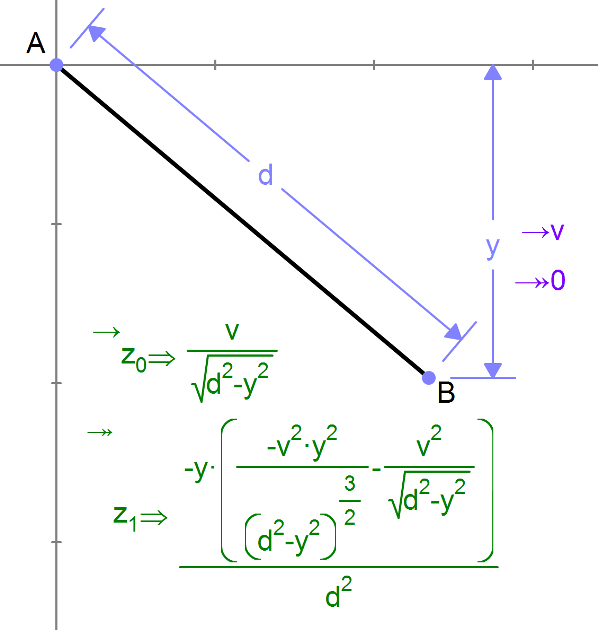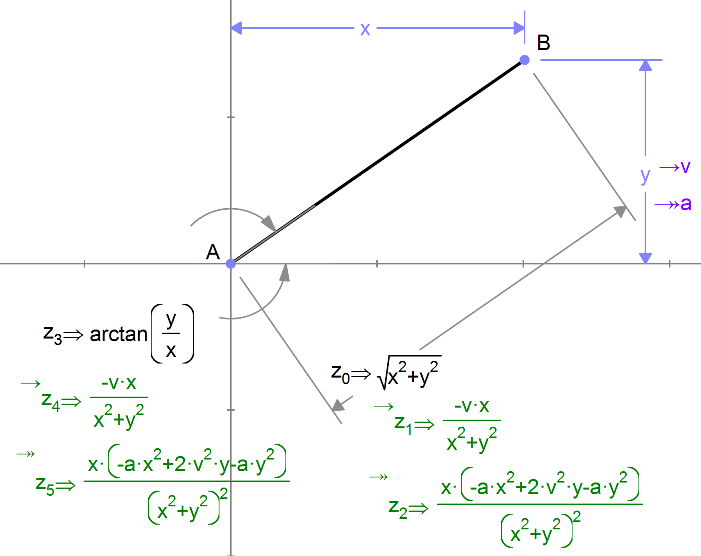# Kinematics Examples

### Pendulum

Here is a model of a pendulum with given angular velocity (applied to the angle θ.) The velocity and acceleration of the point B are measured.### Angular Velocity

Here is the angular velocity and acceleration of line AB.### Relative Velocity

We can also measure the velocity and acceleration of an output measurement (distance or angle). Here we look at a particle B which is moving vertically with velocity v and acceleration a. We measure its distance from the origin and the angle it makes with the x axis. To measure the apparent velocity and acceleration to an observer at A, we measure the velocity and acceleration of the distance.